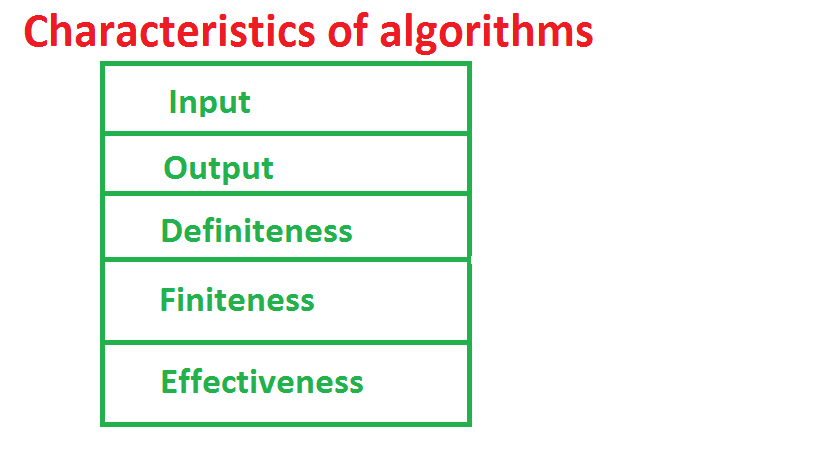Home ComputerAlgorithm Design and Analysis Procedures and Algorithms Introduction

# Procedures and Algorithms Introduction

901 views

### Introduction to Algorithm:

Procedure means method of doing something. Generally procedures and algorithms both means same.

Algorithm is derived from Persian mathematician Musa Al-Khwarizmi, Who is also the father of algebra. Algorithm and data together constitute a program. So good algorithm designing is crucial for robust and efficient programs. An error in designing algorithm can lead to failure in implementing program. Algorithm is answer for many ‘How ‘ questions. This means Algorithms answer to the questions related to method of doing something or solving some problem.

Thus an algorithm is set of finite number of steps that should be followed in order to solve a particular problem. Algorithm is step by step solution of some problem. Generally there are no specific rules to write an algorithm but ultimate goal of algorithm is to tell other about procedure of doing some task.

### Characteristics of Algorithm:Fig: Characteristics Of Algorithm

1. Input: Algorithms can take some input which can be manipulated during the implementation of algorithm. Algorithm can take zero, one or more than one input .
2. Output: Algorithm gives some output on the completion of steps. Every algorithm produce some output as solution. Output is ultimate goal of an algorithm.
3. Definiteness:   The steps in the algorithms should be clear and unambiguous. Every steps in algorithm should not have confusing meaning and should be strictly specified.
4. Finiteness: No of steps in the algorithms should be finite. Algorithm must have countable number of steps so that algorithm can terminate. Otherwise we cannot expect output from algorithm.
5. Effectiveness: Each and every step in algorithm should be simple , basic and meaningful. Unnecessary steps should be omitted. Every step should be effective means that each step should perform some action like input, process or output. Unwanted steps make algorithm long and complex.

We will present some examples and pseudo code.

0 comment

## Procedures and Algorithms Introduction

by Log Raj time to read: 1 min